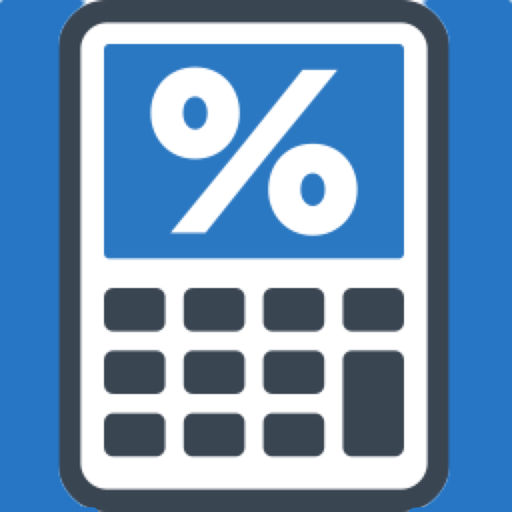Easy Percentage Calculator# Easy Percentage Calculator

Free
0

0 Ratings

Release Date

2016-05-28

Size

9.0 MB

### Screenshots for iPhone

iPhone
Easy Percentage Calculator Description
Our calculator has made it easier than ever before to calculate any percentage you need.
You don't have to use any formulas – you just type in the numbers and calculate.
Examples:
How much is 25% of 110? Or what is the percentage for 11 from 20?
You can use our calculator not only for easy and everyday calculations, but also for complex ones.
Here is the full list of calculations our calculator can help you with:
- What is the percentage for 10 from 100?
- How much is 10% of 100?
- What is the total amount if 10 equals 20% of the total amount?
- What is the change in percentage if the amount increases from 10 to 20?
- How much is 10 times 20%?
- How much is 10 divided by 20%?
- What is the amount if you increase 10 by 20%?
- What is the amount if you decrease 10 by 20%?
Easy Percentage Calculator 2.0 Update
2016-05-28 Version History
We have completely revamped our calculator.
We added more than 6 new formulas.
We translated the calculator into German, French, Spanish, Italian and Russian.
More
Price:
Free
Version:
2.0
Size:
9.0 MB
Release Date:
2016-05-28
Developer:
RedVok Software
Language:
English French German Italian Russian Spanish
More
You May Also Like